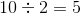# ISEE Middle Level Math : How to find the square root

## Example Questions

### Example Question #81 : Squares / Square Roots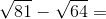Explanation:

First, find the square roots: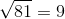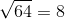Then, solve: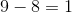### Example Question #82 : Squares / Square Roots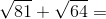Explanation:

First, find the square roots:Then, solve: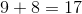### Example Question #83 : Squares / Square Roots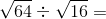Explanation:

First, find the square roots: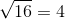Then, solve: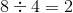### Example Question #84 : Squares / Square Roots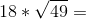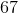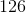Explanation:

First, find the square root: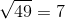Then, solve: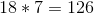### Example Question #85 : Squares / Square Roots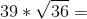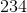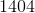Explanation:

First, find the square root: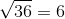Then, solve: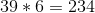### Example Question #86 : Squares / Square Roots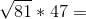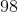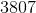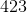Explanation:

First, find the square root:Then, solve: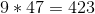### Example Question #87 : Squares / Square Roots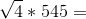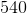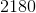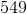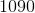Explanation:

First, find the square root: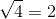Then, solve: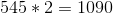### Example Question #88 : Squares / Square Roots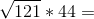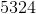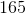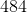Explanation:

First, find the square root: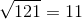Then, solve: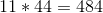### Example Question #89 : Squares / Square Roots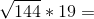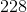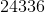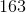Explanation:

First, find the square root: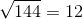Then, solve: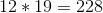### Example Question #90 : Squares / Square Roots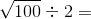Explanation:

First, find the square root: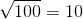Then, solve: